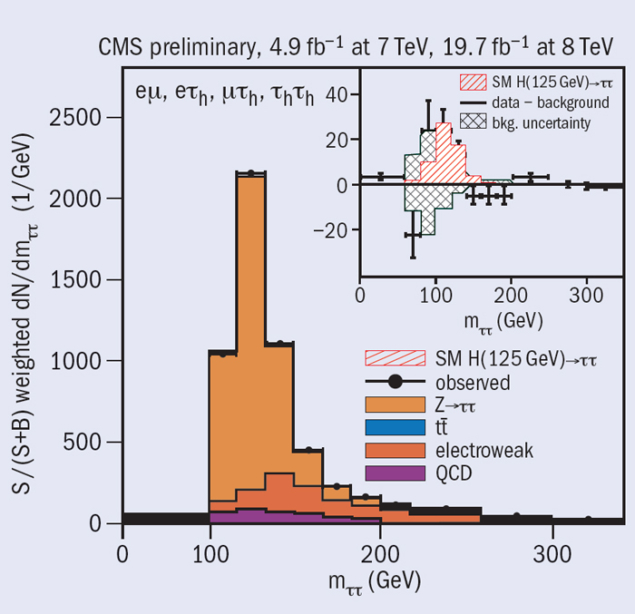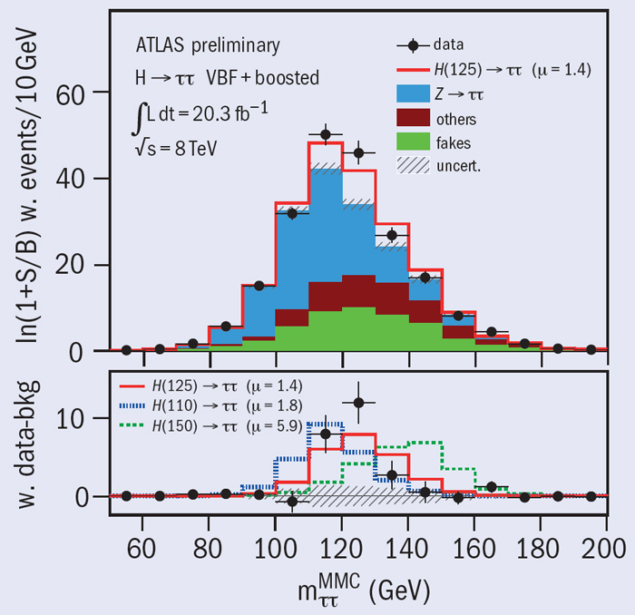# ATLAS and CMS observe Higgs-boson decays to fermions

22 January 2014

Last year, the ATLAS and CMS collaborations confirmed that the new boson found in 2012 was indeed a Higgs boson with a mass around 125 GeV. The discovery relied on the observation of decays to pairs of bosons, namely photons, Ws and Zs – the carriers of the electromagnetic and weak forces – and provided strong support for the idea that the Brout–Englert–Higgs mechanism is responsible for the mass of the W and Z bosons, while leaving the photon massless. Now, with the full LHC data sets from 2011 and 2012, the two collaborations have turned their attention to testing whether the Higgs field also gives mass to fermions, i.e. quarks and leptons.

The CMS collaboration announced its first results on the coupling of the recently discovered Higgs boson to fermion pairs at the Rencontres de Moriond conference, in March 2013. At the time, the search for the Higgs-boson decays to b b and τ+τ had yielded evidence with a combined significance of 3.4σ for the Higgs coupling to third-generation fermions.

Now, the updated search for decays to τ+τ, based on an improved analysis of 4.9 fb−1 of LHC data collected at a collision energy of 7 TeV in 2011 and 19.7 fb−1 collected at 8 TeV in 2012, has revealed a 3.4σ excess at the mass of the Higgs boson in this channel alone. Taken together with the 2.1σ excess found in the earlier searches by CMS for b decays, this gives a combined significance of 4.0σ for the two channels, compared with an expectation of 4.2σ for a Standard Model Higgs boson. The observed rate for Higgs production with subsequent decay into b quarks or τ leptons divided by the expected rate for a Standard Model Higgs gives the ratio μ = 0.90±0.26, which suggests that the particle does indeed behave like a Standard Model Higgs boson.Fig. 1. Weighted mass spectrum for the τ+τ– system in CMS. The inset shows a background-subtracted view of the spectrum with an excess around the mass of the discovered Higgs boson.

By contrast, the search for decays of the Higgs boson to μ+μ yields no signal, just as expected from the fact that the μ has nearly 17 times less mass than the τ, making the decays of the Higgs to μ+μ some 290 times less frequent than those to τ+τ. Likewise, the search for the Higgs-boson decay into a pair of electrons – which should be even more rare, given that the electron is 200 times lighter than the muon – returned empty handed. From this, it can be inferred that the couplings of the Higgs boson to leptons of different generations are not universal, in contrast to, for example, the couplings of the Z bosons, which do not distinguish between lepton flavours.

These measurements used the full capabilities of the CMS experiment, combining information from all of the detector’s components to reconstruct and measure the energy of each individual particle emerging from the proton–proton collision, via a “particle flow” algorithm. This technique allows τ decays to be identified efficiently, while rejecting the background from “jets” of particles originating from quarks and gluons. The precise charged-particle tracking of CMS helps identify jets coming from b quarks. In the case of Higgs-boson decays to τ+τ, the data have a strong peak at a τ+τ mass corresponding to that of the Z boson, which is produced much more copiously than Higgs bosons. The analysis was developed and optimized in a “blinded” way, i.e. not looking at the signal in the data, to avoid introducing a bias.

By carefully measuring and controlling this background, a clear signal from Higgs decays remains after subtracting the background (figure 1).

Armed with an array of techniques and decay modes with which to study the Higgs boson, CMS will continue to measure its properties ever more precisely – using present and future data – and to search for additional new particles, including possible cousins of the Higgs boson.

The ATLAS collaboration presented its first results on fermionic decays of the Higgs boson using the full 2012 data set in the di-muon and b b decay channels at the winter and summer conferences in 2013, respectively. However, the results did not yet establish the fermionic decays expected from a Standard Model Higgs boson. ATLAS has now found strong evidence for Higgs decays to fermions, using 20.3 fb−1 of LHC data taken at a proton collision energy of 8 TeV in the centre of mass. This is an important test of the Standard Model, which predicts such decays.

Branching ratios for the Standard Model Higgs boson are predicted to scale with the mass-squared of the decay products. Hence the two fermionic final states expected to be most abundant are b b quark pairs and τ+τ lepton pairs, the most massive of the fermions to which the Higgs boson can decay.Fig. 2. The invariant mass of the τ+τ– pair in ATLAS. The excess of data events (black dots) is consistent with the presence of a Higgs boson at 125 GeV, indicated by the red line. The main remaining background is Z → τ+τ–. Background contributions with τ+τ– invariant mass away from 125 GeV are suppressed by the analysis.

ATLAS has looked for all possible τ+τ decay channels, namely to two leptons, to one lepton plus hadrons and to hadrons only. The analysis was optimized to test whether a 125 GeV Higgs boson decays to a τ+τ pair and suppresses contributions that have a τ+τ invariant mass away from 125 GeV, including the Z → τ+τ background, although the main remaining background is still Z → τ+τ. Thanks to the detector’s powerful lepton identification capabilities and the application of sophisticated analysis methods, the contamination from backgrounds to the τ+τ final state could be reduced greatly. Remaining backgrounds were estimated directly from the data, or taken from simulation with their rates normalized to data in signal-free control regions. To avoid any possible bias, the analysis was developed and optimized without looking at the signal in the data (blinded).

After “unblinding”, ATLAS observes an excess of events (figure 2) in a region consistent with the previously measured mass of the Higgs boson (125 GeV) and a statistical significance of 4.1σ, against 3.2σ expected. The ratio of the observed signal to that expected for a Standard Model Higgs decaying to a τ+τ pair yields μ = 1.4+0.4–0.5, which is compatible with one.

ATLAS also searched for the decay of a Higgs boson to a muon pair. Because the Higgs boson couples to mass and the mass of the muon is 17 times lower than that of the τ, the H → μ+μ rate is expected to be around 290 times lower than that of H → τ+τ. The absence of a signal in the ATLAS μ+μ search, setting an upper limit of 9.8 times the H → μ+μ rate predicted by the Standard Model, therefore provides strong evidence that the Higgs boson does not decay to leptons in a flavour-blind way, but favours decays to heavy leptons, as predicted by the Standard Model.

These results, which are derived from the LHC’s first run, are so far compatible with the Standard Model predictions. The broad physics programme of ATLAS, which includes precision measurements of the properties of the Higgs boson, will continue to test the Standard Model in the years to come.

These results from the two collaborations now establish the coupling of the Higgs bosons to fermions. More data will allow testing the Higgs couplings at a deeper level, where other models for physics beyond the Standard Model predict differences. The next LHC run, which begins in 2015, is expected to produce several times the existing data sample. In addition, the proton collisions will be at higher energies, producing Higgs bosons at higher rates.